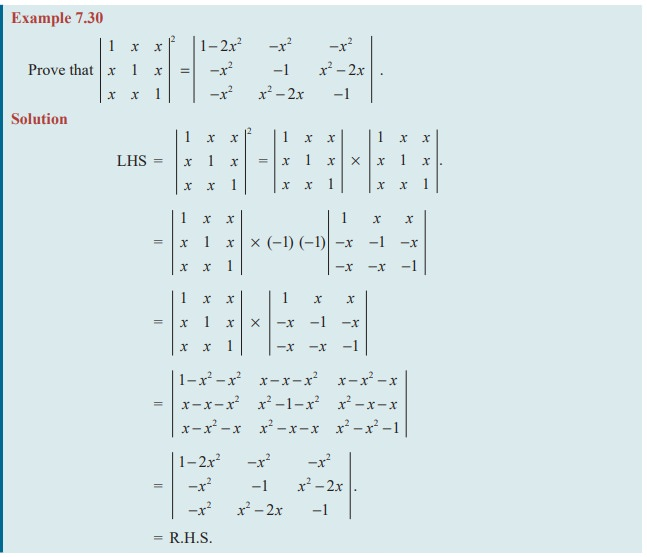Home | | Maths 11th std | Product of Determinants

# Product of Determinants

The process of interchanging the rows and columns will not affect the value of the determinant (by Property 1).

Product of Determinants

While multiplying two matrices “row-by-column” rule alone can be followed. The process of interchanging the rows and columns will not affect the value of the determinant (by Property 1). Therefore we can also adopt the following procedures for multiplication of two determinants.

(i) Row by column multiplication rule

(ii) Row by row multiplication rule

(iii) Column by column multiplication rule

(iv) Column by row multiplication rule

Note 7.11

(i) If A and B are square matrices of the same order n, then | AB | = | A | | B | holds.

(ii) In matrices, although AB ≠ BA in general, we do have | AB | = | BA | always.Tags : Definition, Solved Example Problems , 11th Mathematics : UNIT 7 : Matrices and Determinants
Study Material, Lecturing Notes, Assignment, Reference, Wiki description explanation, brief detail
11th Mathematics : UNIT 7 : Matrices and Determinants : Product of Determinants | Definition, Solved Example Problems